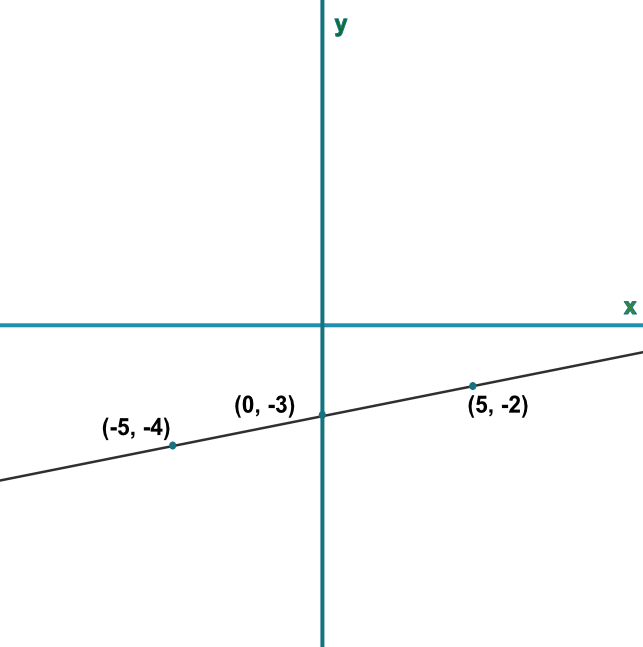Interpret Graphs of Linear Functions

## Interpret Graphs of Linear Functions

A sketch of a function will show the x and y axes and a minimum amount of data, such as where the function cross the x- and y-axes. When more than one function is plotted on the same graph, the different functions must be identified. A scale does not need to be provided:

The equation of a linear function can be determined from a sketch by determining the gradient and intercept points.

## Example 1

There is a piece of information missing from the sketch, below.

What is the missing information?Sketches should contain axis, coordinates of crossing and turning points, and the equation of the line.

Answer: The equation, or function, of the line.

## Example 2

What is the value of the missing piece of information?

A linear function, so the form is y=mx+c

Find the gradient, m

From (0, -3), the gradient goes up 1 for 5 along = frac(1)(5) = 0.2

The intercept c is at (0, 03)

Substitute m and c into the equation for y=0.2x-3

Check using one of the coordinates -4 = 0.2(-5) - 3 ✔

Answer: y=0.2x-3